﻿ Predictability of Phosphorus Removal Based on Simultaneously Removed Sulphur and Mass-Input of Iron Ore Leached in Potassium Hydroxide

### Predictability of Phosphorus Removal Based on Simultaneously Removed Sulphur and Mass-Input of Iron ...

C. I. Nwoye, P. O. Offor, N. E. Idenyi, S. O. Nwakpa, A.O. AgboOPEN ACCESSPEER-REVIEWED

## Predictability of Phosphorus Removal Based on Simultaneously Removed Sulphur and Mass-Input of Iron Ore Leached in Potassium Hydroxide

C. I. Nwoye1,, P. O. Offor2, N. E. Idenyi3, S. O. Nwakpa1, A.O. Agbo4

1Department of Metallurgical and Materials Engineering, Nnamdi Azikiwe University, Awka, Nigeria

2Department of Metallurgical and Materials Engineering, University of Nigeria, Nsukka, Nigeria

3Department of Industrial Physics, Ebonyi State, Abakiliki, Nigeria

4Department of Metallurgical and Materials Engineering, Enugu State University of Science & Technology, Enugu Nigeria

### Abstract

This paper presents the predictability of phosphorus removal based on simultaneously removed sulphur and mass-input of iron ore leached in potassium hydroxide. The reaction vessel containing the solution and ore was heated at a temperature of 600°C for 10minutes. The model; P = 61.8477 x 10-3μ - 2.27S shows that the predicted concentration of removed phosphorus, is dependent on the mass-input of iron oxide ore and the concentration of sulphur simultaneously removed during the reduction process. The validity of the model is rooted in the expression k1P = (2μ – k2S) where both sides of the expression are correspondingly equal. The maximum deviation of the model-predicted (D-Model) concentrations of removed phosphorus (during the beneficiation process) from the concentrations obtained from experiment is less than 3.6861%. This translates to confidence level of over 96%. The concentrations of phosphorus removed per unit mass-input of iron oxide ore as obtained from experiment (ExD), derived model (D-Model) and regression model (R-Model) are 1.01, 1.1549 and 0.87 mg kg-1 g-1 respectively. Similarly, the concentrations of phosphorus removed per unit concentration of sulphur simultaneously removed as obtained from experiment, D-Model and R-Model are 1.8843, 2.1546 and 1.6231 mg kg-1 (mg kg-1)-1 respectively. The standard errors in D-Model prediction of the removed phosphorus concentration for each value of simultaneously removed sulphur concentration is 0.0167 compared to ExD (0.6439) and R-Model (2.05 x 10-5). The F-test result between D-Model and R-Model based on removed sulphur concentration is 0.6926, and 0.7633 between D-Model and experimental results. F-test result between R-Models (based on ore mass-input and removed sulphur concentration) was evaluated as 0.9933. D-Model predicted values agree with the experiment that the concentration of phosphorus removed is ≈ 2.3 times higher than the simultaneously removed sulphur concentration.

### At a glance: Figures

12345
Prev Next

• Nwoye, C. I., et al. "Predictability of Phosphorus Removal Based on Simultaneously Removed Sulphur and Mass-Input of Iron Ore Leached in Potassium Hydroxide." Physics and Materials Chemistry 1.2 (2013): 27-33.
• Nwoye, C. I. , Offor, P. O. , Idenyi, N. E. , Nwakpa, S. O. , & Agbo, A. (2013). Predictability of Phosphorus Removal Based on Simultaneously Removed Sulphur and Mass-Input of Iron Ore Leached in Potassium Hydroxide. Physics and Materials Chemistry, 1(2), 27-33.
• Nwoye, C. I., P. O. Offor, N. E. Idenyi, S. O. Nwakpa, and A.O. Agbo. "Predictability of Phosphorus Removal Based on Simultaneously Removed Sulphur and Mass-Input of Iron Ore Leached in Potassium Hydroxide." Physics and Materials Chemistry 1, no. 2 (2013): 27-33.

 Import into BibTeX Import into EndNote Import into RefMan Import into RefWorks

### 1. Introduction

The adverse effects of high phosphorus content in iron ore and associated finished products such as iron and steel have led to punitive legislation across the world aimed at discouraging importation of iron ores with high phosphorus content. In addition, the development of an economical dephosphorisation process is critical for the future success of iron ore, iron and steel making industry.

Effective dephosphorisation of Western Australian iron has been demonstrated . In this research work, sulphuric acid was chosen as the leachant on the basis of its availability and low cost. The iron ore sample used in this study typically contained 0.126% phosphorus. After roasting at 1250°C, lump ore (P80 5.6 mm), pellet 1 (grinding to 100%, -1.5 mm before pelletisation) and pellet 2 (grinding to 100%, -0.15 mm before pelletisation) were leached in solutions with different sulphuric acid concentrations. After leaching for 5 hours at 60°C in 0.1 M sulphuric acid solution, 67.2%, 69.0% and 68. 7% of the phosphorus was leached from the above three samples, respectively. The phosphorus content was reduced from 0.126% to 0.044%, 0.055% and 0.042% respectively. The dissolution of iron during leaching was negligible. The optimum sulphuric acid concentration was 0. 1 M in terms of acid cost and iron loss. The acid consumption cost was found to be as low as \$A 0.47/tonne .

A process has been proposed  for the treatment of apatite concentrate obtained from the dephosphorization of iron ore. In this process, the apatite is acidulated by HNO3 to produce high-purity H3PO4. The crude acid obtained was shown  to be at least equivalent to, if not better than, the typical wet-process acid. This study indicates that H3PO4 was purified by extraction and concentrated by evaporation. The spent HNO3 was regenerated by H2SO4 to precipitate gypsum. Rare earths dissolved during the apatite acidulation are recovered by precipitation with oxalic acid. An improvement measure to this process  was considered to be: integration of the process  with the processing of the solutions obtained from the direct leaching of iron ore (the phosphorus removal) with HNO3. It was discovered  that the integrated process  is more economical than the single treatment and also it offers the flexibility of producing both sinter-fines and pellets to meet demand in steel manufacture.

A method  for dephosphorizing from phosphor-containing iron ore has been put in place, wherein phosphor-containing iron ore is broken to the granularity of less than 0.074mm and mixed with iron pyrite prebroken to the granularity of less than 0.074mm based on mass percent of 5%-20%. The mass concentration of ore slurry is adjusted to 10%-20% by the aphosphorosis 9K culture and pH of original ore slurry is in the range of 1.5 to 3.5. The inoculating ferrous and sulfur oxide bacilli lysate of 2% -10% mass percent in which bacteria is cultured by 9K culture beforehand to reach exponential phase of growth in ore slurry, extracting dephosphorization, solid-liquid separation after 30 -45 days, and the solid is iron ore concentrate. The invention  is suitable for direct-extracting and dephosphorizing from each phosphor-containing iron ore microbe and the ore dephosphorizing yield can exceed 80%. The content of phosphorus satisfies the requirement of blast furnace ironmaking materials, and having comprehensive utilization of iron pyrite. The process  provides a reliable technical support for high phosphorus iron ore in countries with low cost and good dephosphorization.

Further biological processes for phosphorus removal has been evaluated based on the use of several types of fungi, some being oxalic acid producing. Recently, results  were presented following a study on the use of Aspergillus niger and their cultural filtrates for removing phosphorus from Agbaja (Nigeria) iron oxide ore. The results of this work  show that phosphorus removal efficiencies at the end of the 49 days of the leaching process are 81, 63 and 68% for 5, 100 and 250 mesh grain sizes respectively.

Experimental processes and derived models [5, 6, 7] have shown that hydrometallurgical dephosphorization of iron oxide ore during leaching, using oxalic acid solution, is highly dependent on the final pH of the leaching solution which varies time and other factor such as initial solution pH, initial leaching temperature, mass-input of the iron oxide ore and ore mineralogy etc.

Results  obtained from experiment and derived models have indicated that the removal of phosphorus during leaching of iron oxide ore in oxalic acid solution is also achievable based the mass-input of iron oxide ore and the final solution pH. This is because varied mass-inputs of iron oxide ore results to varied final solution pH.

Model for predictive analysis of the concentration of phosphorus removed during leaching of iron oxide ore in sulphuric acid solution has been derived . In this work  both experimental results and results from the predicted model  indicate that phosphorus removal from the iron oxide ore is dependent on the initial and final pH of the leaching solution. This is because the final pH of the leaching solution is greatly determined by the initial pH which initially has a specified mole of hydrogen ion.

It was also found using a derived model  that the concentration of removed phosphorus during leaching of iron oxide ore in oxalic acid could be evaluated and predicted depending on the leaching temperature. The model indicates that at a leaching temperature range 45-70°C, the maximum deviation of the model-predicted concentration of removed phosphorus from the corresponding experimental values is less than 29%.

The present work is predictability of phosphorus removal based on simultaneously removed sulphur and mass-input of iron ore (from Itakpe, Nigeria) leached in potassium hydroxide.

### 2. Model

The solid phase (ore) is assumed to be stationary, contains the un-leached iron remaining in the ore. It is strongly believed that hydrogen peroxide gas produced from the reaction between KOH and Fe2O3 decomposed to produce oxygen gas (in agreement with past findings ) which oxidized phosphorus and sulphur, hence removing them from the ore in the form of P2O5 and SO2 respectively. Equations (1-4) show this.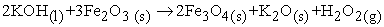(1)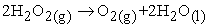(2)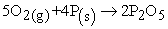(3)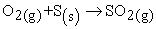(4)
2.1. Model Formulation

Experimental data obtained from research work  were used for the model formulation as shown in Table 1. Computational analysis of these experimental data in Table 1, resulted to Table 2 which indicates that;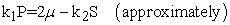(5)

Introducing the values of k1 and k2 into equation (5) reduces it to;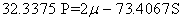(6)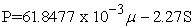(7)

Where

(μ) = Mass-input of iron oxide ore during the leaching process (g)

S = Concentration of sulphur removed during leaching of the iron oxide ore using KOH (mg kg-1)

P = Concentration of phosphorus removed during leaching of the iron oxide ore using KOH (mg kg-1)

k1 = 32.3375; k2 = 73.4067

The constants k1 and k2 are Phosphorus Leachibility Resistance (PLR) and Sulphur Leachibility Resistance (SLR) in KOH solution at 600°C respectively (determined using C-NIKBRAN ). These constants give the resistance to leaching out of phosphorus and sulphur (in KOH solution at 600°C) from iron oxide ore due to the presence of other elements or compounds which respectively binds the phosphorus and sulphur. Furthermore, these constants are dependent on the various degrees at which phosphorus and sulphur respectively interweave with the other compounds present in the iron ore oxide.

#### Table 1. Variation of the concentration of phosphorus removed with concentration of sulphur removed and mass input of iron oxide ore 

2.2. Boundary and Initial Condition

Leaching solution of potassium hydroxide was added to a cylindrical flask 30cm high containing iron oxide ore. The leaching solution is stationary i.e (non-flowing). The flask is assumed to be initially free of attached bacteria. Initially, atmospheric levels of oxygen are assumed. Varying masses μ (2-8g) of iron oxide ore were used as outlined in Table 1. The leaching time, leaching temperature, ore grain size, and potassium hydroxide concentration: 10 mins., 600°C, 60μm and 0.112mole/litre were used respectively. These and other process conditions are as stated in the experimental technique .

The boundary conditions are: atmospheric levels of oxygen (since the cylinder was open at the top) at the top and bottom of the ore particles in the liquid and gas phases respectively. At the bottom of the particles, a zero gradient for the liquid scalar are assumed and also for the gas phase at the top of the particles. The leaching solution is stationary. The sides of the particles are taken to be symmetries .

### 3. Results and Discussion

3.1. Model Validation

The derived model is equation (7). Computational analysis of the experimental data in Table 1 gave rise to Table 2.

It was found that the validity of the model is rooted in the expression k1P = 2μ - k2S where both sides of the expression are correspondingly approximately almost equal. Table 2 also agrees with equation (6) following the values of k1P and 2μ - k2S evaluated from Table 1 as a result of the corresponding computational analysis.

#### Table 2. Variation of k1P with (2μ – k2S)

3.2. Statistical Analysis

In addition, the model was validated by comparing results of standard error (STEYX), correlation (CORREL) and F-test obtained from experiment, derived model and regression model which was applied as a standard model. All these were evaluated using Microsoft Excel .

3.3. Standard Error (STEYX)

This gives the error incurred in predicting the concentration of phosphorus removed for each value of the ore mass-input and concentration of sulphur simultaneously removed. This was calculated using Microsoft Excel . Table 3 shows the standard errors incurred by the derived model (D-Model), experiment (ExD) and regression model (R-Model) in predicting the concentration of removed phosphorus for each values of ore mass-input and removed sulphur concentration.

#### Table 3. Comparison of the standard errors evaluated from ExD, D-Model and R-Model predicted results based on ore mass-input and simultaneously removed sulphur concentration

3.4. Correlation (CORREL)

Correlation coefficient determines the relationship between two properties. The correlations between the removed phosphorus concentration and ore mass-input Figure 1, Figure 2, and Figure 3, as well as between the removed phosphorus concentration and simultaneously removed sulphur concentration Figure 4, Figure 5, and Figure 6, as obtained from ExD, D-Model and R-Model respectively are shown in Table 4. These values were evaluated using Microsoft Excel :

3.5. F-test

This is a one tailed probability that the variance between two data sets is not significantly different. The probability that the variance between derived model (D-Model) and regression model (R-Model) as well as between derived model and experiment is shown in Table 5. These values were evaluated using Microsoft Excel .

#### Table 4. Comparison of the correlations evaluated from ExD, D-Model and R-Model predicted results based on ore mass-input and simultaneously removed sulphur concentration

Figure 1. Variation of mass-input of iron oxide ore with the concentration of phosphorus removed as obtained from experiment 
Figure 4. Variation of the concentration of phosphorus removed with concentration of removed sulphur as obtained from experiment 
Figure 5. Variation of the concentration of phosphorus removed with concentration of removed sulphur as predicted by D-Model
Figure 6. Variation of the concentration of phosphorus removed with concentration of removed sulphur as predicted by R-Model

Furthermore, F-test carried out shows that the probability that the variance between R-Model predicted results based on ore mass-input and R-Model predicted results based on removed sulphur concentration is not significantly different is 0.9933. This shows that the values from R-Model (based on ore mass-input and removed sulphur concentration) are approximately equal.

#### Table 5. Comparison of the F-test results evaluated from ExD, D-Model and R-Model predicted results based on ore mass-input and simultaneously removed sulphur concentration

3.6. Computational Analysis

Determination of the Concentration of Phosphorus Removed per Unit Mass of Iron Oxide Ore Added

Concentration of phosphorus removed (during the thermo-leaching of the iron ore) per unit mass of iron oxide ore Pμ (mg kg-1 g -1) is calculated from the equation;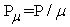(8)

Therefore, a plot of concentration of phosphorus removed against mass of iron oxide ore added (as in Figure 1) gives a slope, S at points (49.95, 8) and (44.9, 3) following their substitution into the mathematical expression;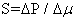(9)

Equation (9) is detailed as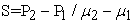(10)

Where

ΔP = Change in the concentrations of phosphorus removed P2, P1 at mass values μ2, μ1. Considering the points (49.95, 8) and (44.9, 3) for (P2, μ2) and (P1, μ1) respectively, and substituting them into equation (10), gives the slope as 1.01 mg kg-1 g-1 which is the concentration of phosphorus removed per unit mass of iron oxide ore added during the actual experimental leaching process. Also a plot (as in Figure 2) using the D-Model predicted results gives a slope. Considering points (50.8757, 8) and (45.1013, 3) for (P2, μ2) and (P1, μ1) respectively and substituting them into equation (10) gives the value of slope, S as 1.1549 mg kg-1 g-1. This is the D-Model predicted concentration of phosphorus removed per unit mass of iron oxide ore used for the leaching process. Similarly, a plot (as in Figure 3) using the R-Model predicted results gives a slope S as 0.87 mg kg-1 g-1 on substituting points (49.175, 8) and (44.825, 3) for (P2, μ2) and (P1, μ1) respectively into equation (10). This is the R-Model predicted concentration of phosphorus removed per unit mass of iron oxide ore used for the leaching process. A comparison of these three values of removed phosphorus concentration per unit mass of iron oxide ore used for the leaching process shows proximate agreement and invariably a very high degree of validity for the D-Model.

Determination of the Concentration of Phosphorus Removed per Unit Concentration of removed sulphur

Similarly, the concentration of phosphorus removed (during the thermo-leaching of the iron ore) per unit concentration of removed sulphur Ps; mg kg-1 (mg kg)-1 is calculated from the equation;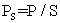(11)

A plot of concentration of phosphorus removed against concentration of simultaneously removed sulphur (as in Figure 4) using experimental results gives a slope, Se = 1.8843 mg kg-1 (mg kg-1)-1 at points (49.95, 22.63) and (44.9, 19.95) for (P2, S2) and (P1, S1) respectively following their substitution into the mathematical expression;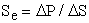(12)

which is detailed as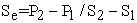(13)

Where

ΔP = Change in the concentrations of phosphorus removed P2, P1 at two concentration values of removed sulphur S2, S1. This is the concentration of phosphorus removed per unit concentration of simultaneously removed sulphur during the actual experimental treatment of the iron oxide ore.

A plot (as in Figure 5) using D-Model predicted results gives a slope, Se = 2.1546 mg kg-1 (mg kg)-1 at points (50.8757, 22.63) and (45.1013, 19.95) for (P2, S2) and (P1, S1) respectively following their substitution into equation (13). This is the D-Model predicted concentration of phosphorus removed per unit concentration of simultaneously removed sulphur. Similarly, a plot (as in Figure 6) using the R-Model predicted results gives a slope S as 1.6231 mg kg-1 (mg kg)-1 on substituting points (49.175, 22.63) and (44.825, 19.95) for (P2, S2) and (P1, S1) respectively into equation (13). This is the R-Model predicted concentration of phosphorus removed per unit mass of iron oxide ore used for the leaching process. A comparison of these three values of removed phosphorus concentration per unit concentration of simultaneously removed sulphur shows proximate agreement. This also indicates a very high degree of validity for the D-Model.

3.7. Graphical Analysis

Comparison of Figure 7, Figure 8, Figure 9, Figure 10, show that both values of the concentration of phosphorus removed obtained from the experiment  (line ExD) and the derived model (line D-Model) in relation to both the mass of iron oxide ore (added) and concentration of sulphur simultaneously removed are generally quite close hence depicting proximate agreement and validity of the model.

Figure 7. Comparison of the concentrations of phosphorus removed in relation to mass-input of iron oxide ore as obtained from experiment  and D-Model
Figure 8. Comparison of the concentrations of phosphorus removed in relation to the concentration of sulphur simultaneously removed as obtained from experiment  and D-Model
Figure 9. Comparison of the concentrations of phosphorus removed in relation to mass-input of iron oxide ore as obtained from experiment , D-Model and R-Model.
Figure 10. Comparison of the concentrations of phosphorus removed in relation to the concentration of sulphur simultaneously removed as obtained from experiment , D-Model and R-Model

D-Model predicted values as shown in Figure 11 agree with the corresponding experimental values that the concentration of phosphorus removed is ≈ 2.3 times higher than the simultaneously removed sulphur concentration. This is real since it agrees with the fact that leachibility resistance to sulphur removal is ≈ 2.3 times higher than that for phosphorus removal. Mathematically, this implies that SLR (k2) ≈ 2.3 PLR (k1).

Figure 11. Showing D-Model predicted concentration of removed phosphorus as ≈ 2.3 times higher than the simultaneously removed sulphur concentration
3.8. Deviational Analysis

The formulated model was also validated by direct analysis and comparison of model-predicted P values and those obtained from experiment  for equality or near equality. Analysis and comparison between these P values reveal deviation of model-predicted P values from those of the experiment. This is believed to be due to the fact that the surface properties of the ore and the physiochemical interactions between the ore and leaching solution which were found to play vital roles during the leaching process  were not considered during the model formulation. This necessitated the introduction of correction factor, to bring the model-predicted P values to those of the experimental values.

Deviation (Dv) (%) of model-predicted P values from the experimental P values is given by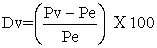(14)

Where

Pv = Model-predicted concentration of phosphorus removed (mg kg-1)

Pe = Concentration of phosphorus removed as obtained from experiment (mg kg-1)

Correction factor (Cr) is the negative of the deviation i.e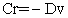(15)

Therefore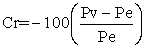(16)

Introduction of the corresponding values of Cf from equation (16) into the D-Model gives exactly the corresponding experimental P values .

Variation of mass-input of iron oxide ore and model-predicted concentration of removed phosphorus with the associated deviations

The maximum deviation of the model-predicted concentration of removed phosphorus, from the corresponding experimental value is 3.6861% which is quite within the acceptable deviation range of experimental results. Figure 12 indicates that the highest and least deviations; 3.6861% and 0.4483% corresponds to the D-Model predicted concentrations of removed phosphorus: 48.5251 and 45.1013 mg kg-1 respectively. Comparison of Figure 12, and Figure 13 shows that these values correspond to the mass-input of iron oxide ore: 6 and 3g respectively.

Figure 12. Variation of D-Model predicted concentration of phosphorus removed with its associated deviation from experimental values 
Figure 13. Effect of mass-input of iron oxide ore on the deviation of D-Model predicted concentration of removed phosphorus (from experimental values)
Figure 14. Variation of D-Model predicted concentration of phosphorus removed with its associated correction factor

Variation of the correction factor with the D-Model predicted concentration of phosphorus removed

The computed values of the correction factor as shown in Figure 14 indicate that the highest and least correction factors (-3.6861% and -0.4483%) are same in magnitude and similarly corresponds to the D-Model predicted concentrations of removed phosphorus: 48.5251 and 45.1013 mg kg-1 and the mass-input of iron oxide ore: 6 and 3g respectively.

Comparative analysis of Figure 12, Figure 13, and Figure 14 shows that the values of the correction factor are opposite that of the deviation hence the nature of curves. This is attributed to the fact that correction factor is the negative of the deviation as shown in equations (15) and (16). It is strongly believed that the correction factor takes care of the effects of the surface properties of the ore and the physiochemical interaction between the ore and the leaching solution which (affected experimental results) were not considered during the model formulation.

Phosphorus removed per unit mass of iron oxide ore added during the leaching process was determined following comparison of the concentration of phosphorus removed per unit mass-input of iron oxide ore obtained by calculations involving experimental results as well as derived model.

### 4. Conclusion

The derived model (D-Model) gave an assessment evaluation of removed phosphorus concentration based on simultaneously removed sulphur and mass-input of iron ore leached in potassium hydroxide. The validity of the model is rooted in the expression k1P = (2μ – k2S) where both sides of the expression are correspondingly approximately almost equal. The maximum deviation of the model-predicted (D-Model) concentrations of removed phosphorus (during the beneficiation process) from the concentrations obtained from experiment is less than 3.6861%. This translates to confidence level of over 96%. The concentrations of phosphorus removed per unit mass-input of iron oxide ore as obtained from experiment (ExD), derived model (D-Model) and regression model (R-Model) are 1.01, 1.1549 and 0.87 mg kg-1 g-1 respectively. Similarly, the concentrations of phosphorus removed per unit concentration of sulphur simultaneously removed as obtained from experiment, D-Model and R-Model are 1.8843, 2.1546 and 1.6231 mg kg-1 (mg kg-1)-1 respectively. The standard error in D-Model prediction of the removed phosphorus concentration for each value of simultaneously removed sulphur concentration is 0.0167 compared to ExD (0.6439) and R-Model (2.05 x 10-5). The F-test result between D-Model and R-Model based on removed sulphur concentration is 0.6926, and 0.7633 between D-Model and experimental results. F-test result between R-Models (based on ore mass-input and removed sulphur concentration) was evaluated as 0.9933. D-Model predicted values agree with the experiment that the concentration of phosphorus removed is ≈ 2.3 times higher than the simultaneously removed sulphur concentration.

### References

  Cheng, C. Y., Misra, V. N., Clough, J., Mun, R., 1999. Dephosphorisation of Western Australian iron ore by Hydrometallurgical process. Miner. 12, pp. 1083-1092.In article CrossRef  Zhang, Y. and Muhammed, M., 1990. An integrated process for the treatment of apatite obtained from dephosphorization of iron ore. J. Chem. Tech. & Biotech. 47, pp. 47-60.In article CrossRef  Patent Publication No. 101037724, 2007.In article  Anyakwo, C. N., and Obot, O.W., 2008, Phosphorus Removal from Nigeria`s Agbaja Iron Ore by Aspergillus niger, Inter. Res. J. Eng. Sc. Tech. 5(1), pp. 54-58.In article  Nwoye, C. I., C. N. Mbah., C. C. Nwakwuo., and A. I. Ogbonna., 2010, Model for Quantitative Analysis of Phosphorus Removed during Leaching of Iron Oxide Ore in Oxalic Acid Solution. J. Min. Mater. Charact. Eng. 9(4), pp. 331-341.In article  Nwoye, C. I., 2009, Model for Evaluation of the Concentration of Dissolved Phosphorus during Leaching of Iron Oxide Ore in Oxalic Acid Solution. J. Min. Mater. Charact. Eng. 8(3), pp. 181-188.In article  Nwoye, C. I., Agu, P. C., Mark, U., Ikele, U. S., Mbuka, I. E., and Anyakwo, C. N., 2008, Model for Predicting Phosphorus Removal in Relation to Weight of Iron Oxide Ore and pH during Leaching with Oxalic Acid. Inter. J. Nat. Appl. Sc. 4(3), pp. 292-298.In article  Nwoye, C. I. and Ndlu, S., 2009, Model for Predictive Analysis of the Concentration of Phosphorus Removed during Leaching of Iron Oxide Ore in Sulphuric Acid Solution J. Min. Mater. Charact. Eng. 8(4), pp. 261-270.In article  Nwoye, C. I., 2009, Model for Predicting the Concentration of Phosphorus Removed during Leaching of Iron Oxide Ore in Oxalic Acid Solution. J. Eng. Appl. Sc. (in press).In article  Odesina, I. A., 2003, Essential Chemistry, 1st Edition, Lagos, Macmillan, pp. 169-186.In article  Nwoye, C. I., 2009, SynchroWell Research Work Report, DFM Unit, No 2007146, pp. 35-45.In article  Nwoye, C. I., 2008. C-NIKBRAN; Data Analytical Memory.In article  Microsoft Excel. 2003 version.In article